# NCERT Solutions for Class 8 Maths Chapter 8 Comparing Quantities| PDF Download

On this page, one can find Chapter 8 Comparing Quantities Class 8 Maths NCERT Solutions that is going to help in understanding the key concepts in an better way. You can download PDF of NCERT Solutions for Class 8 Maths Chapter 8 Comparing Quantities through which you'll able to solve the difficult problems in a given in a exercise. There are various concepts given in the Chapter 8 Class 8 Maths NCERT Solutions that will develop your problem solving skills.

By practising more and more questions from the Chapter 8 Comparing Quantities, a student will be able to get more marks in the examinations and recall various questions any time. Also, you can build your own answers for homework and get good marks in the examination.Page No: 119

Exercise 8.1

1. Find the ratio of the following:
(a) Speed of a cycle 15 km per hour to the speed of scooter 30 km per hour.
(b) 5 m to 10 km
(c) 50 paise to Rs. 5

Solution

(a) Speed of cycle = 15 km/hr
Speed of scooter = 30 km/hr
Hence ratio of speed of cycle to that of scooter = 15 : 30 = 15/30 = 1/2= 1 : 2

(b) ∵ 1 km = 1000 m
∴ 10 km = 10×1000 = 10000 m
∴ Ratio = 5m/10000m = 1/2000 = 1 : 2000

(c) ∵ Rs 1 = 100 paise
∴ Rs 5 = 5× 100 = 500 paise
Hence Ratio = 50 paise/ 500 paise = 1/10 = 1 : 10

2. Convert the following ratios to percentages:
(a) 3 : 4
(b) 2 : 3

Solution

(a) Percentage of 3 : 4 = 3/4 ×100 % = 75%
(b) Percentage of 2 : 3 = 2/3 × 100% = 66.2/3%

3. 72% of 25 students are good in mathematics. How many are not good in mathematics?

Solution

Total number of students = 25
Number of good students in mathematics = 72% of 25 = 72/100 ×25 = 18
Number of students not good in mathematics = 25 – 18 = 7
Hence percentage of students not good in mathematics = 7/25 × 100 = 28%

4. A football team won 10 matches out of the total number of matches they played. If their win percentage was 40, then how many matches did they play in all?

Solution

Let total number of matches be x.
According to question,
40% of total matches = 10
⇒ 40% of x = 10
⇒ 40/100 × x = 10
⇒ x = (10×100)/40 = 25
Hence total number of matches are 25.

5. If Chameli had Rs. 600 left after spending 75% of her money, how much money did she have in the beginning?

Solution

Total percentage of money she didn't spent = 100% - 75%=25%
According to question,
25% = 600
1% = 600/25
100% = 600/ 25 × 100
Hence the money in the beginning was Rs 2,400.

6. If 60% people in a city like cricket, 30% like football and the remaining like other games, then what percent of the people like other games? If the total number of people are 50 lakh, find the exact number who like each type of game.

Solution

Number of people who like cricket = 60%
Number of people who like football = 30%
Number of people who like other games = 100% – (60% + 30%) = 10%
Now, Number of people who like cricket = 60% of 50,00,000 = 60/100 × 50,00,000 = 30,00,000
And Number of people who like football = 30% of 50,00,000 = 30/100 × 50,00,000 = 15,00,000
∴ Number of people who like other games = 10% of 50,00,000 = 10/100 × 50,00,000 = 5,00,000
Hence, number of people who like other games are 5 lakh.

Pg No. 125

Exercise 8.2

1. A man got 10% increase in his salary. If his new salary is Rs.1,54,000, find his original salary.

Solution

Let original salary be Rs.100.
Therefore New salary i.e., 10% increase
= 100 + 10 = Rs.110
∵ New salary is Rs.110, when original salary = Rs.100
∴ New salary is Rs.1, when original salary = 100/110
∴ New salary is Rs.1,54,000, when original salary = 100/110 × 154000 = Rs.1,40,000
Hence original salary is Rs. 1,40,000.

2. On Sunday 845 people went to the Zoo. On Monday only 169 people went. What is the percent decrease in the people visiting the Zoo on Monday?

Solution

On Sunday, people went to the Zoo = 845
On Monday, people went to the Zoo = 169
Number of decrease in the people = 845 – 169 = 676
Decrease percent = 676/845 ×100 = 80%
Hence decrease in the people visiting the Zoo is 80%.

3. A shopkeeper buys 80 articles for Rs.2,400 and sells them for a profit of 16%. Find the selling price of one article.

Solution

No. of articles = 80
Cost Price of articles = Rs. 2,400
And Profit = 16%
∵ Cost price of articles is Rs.100, then selling price = 100 + 16 = Rs.116
∴ Cost price of articles is Rs.1, then selling price = 116/100
∴ Cost price of articles is Rs.2400, then selling price = 116/100 × 2400 = Rs.2784
Hence, Selling Price of 80 articles = Rs.2784
Therefore Selling Price of 1 article = 2784/80 = Rs.34.80

4. The cost of an article was Rs.15,500, Rs.450 were spent on its repairs. If it sold for a profit of 15%, find the selling price of the article.

Solution

Here, C.P. = Rs.15,500 and Repair cost = Rs.450
Therefore Total Cost Price = 15500 + 450 = Rs.15,950
Let C.P. be Rs.100, then S.P. = 100 + 15 = Rs.115
When C.P. is Rs.100, then S.P. = Rs.115
∴ When C.P. is Rs.1, then S.P. = 115/100
∴ When C.P. is Rs.15950, then S.P. =115/100 × 15950 = Rs.18,342.50

5. A VCR and TV were bought for Rs.8,000 each. The shopkeeper made a loss of 4% on the VCR and a profit of 8% on the TV. Find the gain or loss percent on the whole transaction.

Solution

Cost price of VCR = Rs.8000 and Cost price of TV = Rs.8000
Total Cost Price of both articles = Rs.8000 + Rs.8000 = Rs. 16,000
Now, VCR is sold at 4% loss.
Let C.P. of each article be Rs.100, then S.P. of VCR = 100 – 4 = Rs.96
When C.P. is Rs.100, then S.P. = Rs.96
∴ When C.P. is Rs.1, then S.P. = 96/100
∴ When C.P. is Rs.8000, then S.P. = 96/100 ×8000 = Rs.7,680
And TV is sold at 8% profit, then S.P. of TV = 100 + 8 = Rs.108
When C.P. is Rs.100, then S.P. = Rs.108
∴ When C.P. is Rs.1, then S.P. = 108/100
∴ When C.P. is Rs.8000, then S.P. = 108/100 × 8000 = Rs.8,640
Then, Total S.P. = Rs.7,680 + Rs.8,640 = Rs. 16,320
Since S.P. >C.P.,
Therefore Profit = S.P. – C.P. = 16320 – 16000 = Rs.320
And Profit% = Profit/ cost price × 100 = 320/16000 × 100 = 2%
Therefore,the shopkeeper had a gain of 2% on the whole transaction.

6. During a sale, a shop offered a discount of 10% on the marked prices of all the items. What would a customer have to pay for a pair of jeans marked at Rs.1450and two shirts marked at Rs.850 each?

Solution

Rate of discount on all items = 10%
Marked Price of a pair of jeans = Rs.1450 and Marked Price of a shirt = Rs.850
Discount on a pair of jeans = (Rate × M.P)/100 = (10×1450)/100 = Rs.145
∴ S.P. of a pair of jeans = Rs.1450 – Rs.145 = Rs.1305
Marked Price of two shirts = 2× 850 = Rs.1700
Discount on two shirts = (Rate × M.P)/100 = (10 × 1700)/100 = Rs.170
∴ S.P. of two shirts = Rs.1700 – Rs.170 = Rs.1530
Therefore the customer had to pay = 1305 + 1530
= Discount on a pair of jeans
= (Rate × M.P)/100
= (10×1450)/100 = Rs.145
∴ S.P. of a pair of jeans = Rs.1450 – Rs.145 = Rs.2,835
Thus,the customer will have to pay Rs.2,835

7. A milkman sold two of his buffaloes for Rs.20,000 each. On one he made a gain of 5% and on the other a loss of 10%. Find his overall gain or loss. (Hint: Find CP of each)

Solution

S.P. of each buffalo = Rs.20,000
S.P. of two buffaloes = 20,000×2 = Rs.40,000
One buffalo is sold at 5% gain.
Let C.P. be Rs.100, then S.P. = 100 + 5 = Rs.105
When S.P. is Rs.105, then C.P. = Rs.100
∴ When S.P. is Rs.1, then C.P. = 100/105
∴ When S.P. is Rs.20,000, then C.P. = 100/105 × 20000 = Rs.19,047.62
Another buffalo is sold at 10% loss.
Let C.P. be Rs.100, then S.P. = 100 – 10 = Rs.90
When S.P. is Rs.90, then C.P. = Rs.100
∴ When S.P. is Rs.1, then C.P. = 100/90
∴ When S.P. is Rs.20,000, then C.P. = 100/90 × 20000 = Rs.22,222.22
Total C.P. = Rs.19,047.62 + Rs.22,222.22 = Rs.41,269.84
Since C.P. >S.P.
Therefore here it is loss.
Loss = C.P. – S.P. = Rs.41,269.84 – Rs. 40,000.00 = Rs.1,269.84
The overall loss of milkman was Rs.1269.84

8. The price of a TV is Rs.13,000. The sales tax charged on it is at the rate of 12%. Find the amount that Vinod will have to pay if he buys it.

Solution

C.P. = Rs.13,000 and S.T. rate = 12%
Let C.P. be Rs.100, then S.P. for purchaser = 100 + 12 = Rs.112
When C.P. is Rs.100, then S.P. = Rs.112
∴ When C.P. is Rs.1, then S.P. = 112/100
∴ When C.P. is Rs.13,000, then S.P. = 112/100 × 13000 = Rs.14,560
He will have to pay Rs.14,560

9. Arun bought a pair of skates at a sale where the discount given was 20%. If the amount he pays is Rs.1,600, find the marked price.

Solution

S.P. = Rs.1,600 and Rate of discount = 20%
Let M.P. be Rs.100, then S.P. for customer = 100 – 20 = Rs.80
When S.P. is Rs.80, then M.P. = Rs.100
∴ When S.P. is Rs.1, then M.P. = 100/80
∴ When S.P. is Rs.1600, then M.P. = 100/ 80 × 1600 = Rs.2,000
Thus, the marked price was Rs. 2,000

10. I purchased a hair-dryer for Rs.5,400 including 8% VAT. Find the price before VAT was added.

Solution

C.P. = Rs.5,400 and Rate of VAT = 8%
Let C.P. without VAT is Rs. 100, then price including VAT = 100 + 8 = Rs.108
When price including VAT is Rs.108, then original price = Rs.100
∴ When price including VAT is Rs.1, then original price = 100/108
∴ When price including VAT is Rs.5400, then original price = 100/108 × 5400 = Rs.5000
Thus, the price of Hair Dryer before the addition of VAT was Rs 5000

Pg No. 133

Exercise 8.3

1. Calculate the amount and compound interest on:
(a) Rs.10,800 for 3 years at 12.1/2% per annum compounded annually.
(b) Rs.18,000 for 2.1/2 years at 10% per annum compounded annually.
(c) Rs.62,500 for 1.1/2 years at 8% per annum compounded annually.
(d) Rs.8,000 for 1 years at 9% per annum compounded half yearly. (You could the year by year calculation using S.I. formula to verify).
(e) Rs.10,000 for 1 years at 8% per annum compounded half yearly.

Solution

(a) Here, Principal (P) = Rs. 10800,
Time(n) = 3 years,
Rate of interest (R) = 12.1/2% = 25/2 %
Amount (A) ==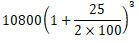=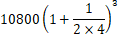=== 10800 × 9/8 × 9/8 × 9/8
= Rs. 15,377.34 (approx.)
Compound Interest (C.I.) = A – P
= Rs. 10800 – Rs. 15377.34 = Rs. 4,577.34

(b) Here, Principal (P) = Rs. 18,000, Time (n) = 2.1/2 years, Rate of interest (R) = 10% p.a.
Amount (A) ===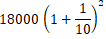= 18000(11/10)2 = 18000 × 11/10 ×11/10
= Rs. 21,780
Interest for 1/2 years on Rs. 21,780 at rate of 10% = (21780×10×1)/100 = Rs. 1,089
Total amount for 2.1/2 years = Rs. 21,780 + Rs. 1089 = Rs. 22,869
Compound Interest (C.I.) = A – P = Rs. 22869 – Rs. 18000 = Rs. 4,869

(c) Here, Principal (P) = Rs. 62500,
Time (n) = 1.1/2 = 3/2 years = 3 years (compounded half yearly)
Rate of interest (R) = 8% = 4% (compounded half yearly)
Amount (A) ==== 62500 (26/25)3
= 62500 × 26/25 × 26/25 × 26/25
= Rs. 70,304
Compound Interest (C.I.) = A – P = Rs. 70304 – Rs. 62500 = Rs. 7,804

(d) Here, Principal (P) = Rs. 8000,
Time (n) = 1 years = 2 years(compounded half yearly)
Rate of interest (R) = 9% = 9/2 % (compounded half yearly)
Amount (A) ==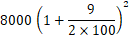=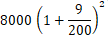= 8000 (209/200)2
= 8000 × 209/200 × 209/200
= Rs. 8,736.20
Compound Interest (C.I.) = A – P = Rs. 8736.20 – Rs. 8000 = Rs. 736.20

(e) Here, Principal (P) = Rs. 10,000,
Time (n) = 1 years = 2 years (compounded half yearly)
Rate of interest (R) = 8% = 4% (compounded half yearly)
Amount (A) ===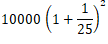= 10000 (26/25)2
= 10000 × 26/26 × 26/25
= Rs. 10,816
Compound Interest (C.I.) = A – P = Rs. 10,816 – Rs. 10,000 = Rs. 816

2. Kamala borrowed Rs.26,400 from a Bank to buy a scooter at a rate of 15% p.a. compounded yearly. What amount will she pay at the end of 2 years and 4 months to clear the loan?
(Hint: Find A for 2 years with interest is compounded yearly and then find SI on the 2nd year amount for 4/12 years).

Solution

Here, Principal (P) = Rs. 26,400,
Time(n) = 2 years 4 months,
Rate of interest (R) = 15% p.a.
Amount for 2 years (A) ===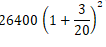= 26400(23/20)2 = 26400 × 23/20 × 23/20
= Rs. 34,914
Interest for 4 months = 4/12 = 1/3 years at the rate of 15% = (34914 × 15×1)/100
= Rs. 1745.70
∴Total amount = Rs. 34,914 + Rs. 1,745.70 = Rs. 36,659.70

3. Fabina borrows Rs.12,500 per annum for 3 years at simple interest and Radha borrows the same amount for the same time period at 10% per annum, compounded annually. Who pays more interest and by how much?

Solution

Here, Principal (P) = Rs.12,500,
Time (T) = 3 years,
Rate of interest (R) = 12% p.a.
Simple Interest for Fabina =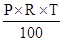= Rs. 4,500
Amount for Radha, P = Rs. 12,500, R = 10% and  n = 3 years
Amount (A)  ==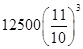== Rs. 16,637.50

C.I. for Radha = A – P
= Rs. 16,637.50 – Rs. 12,500 = Rs. 4,137.50
Thus, Fabina pays more interest = Rs. 4,500 – Rs. 4,137.50 = Rs. 362.50

4. I borrows Rs.12,000 from Jamshed at 6% per annum simple interest for 2 years. Had I borrowed this sum at 6% per annum compound interest, what extra amount would I have to pay?

Solution

Here, Principal (P) = Rs.12,000,
Time (T) = 2 years,
Rate of interest (R) = 6% p.a.
Simple Interest = (P×R×T)/100
= (12000 × 6× 2)/100 = Rs. 1,440
Had he borrowed this sum at 6% p.a., then
Compound Interest =- P
=== 12000(53/50)2 - 12000
= 12000 × 53/50 × 53/50 - 12000
= Rs. 13,483.20 – Rs. 12,000
= Rs. 1,483.20
Difference in both interests = Rs. 1,483.20 – Rs. 1,440.00 = Rs. 43.20
Thus ,the extra amount to be paid is Rs.43.20

5. Vasudevan invested Rs.60,000 at an interest rate of 12% per annum compounded half yearly. What amount would he get:
(i) after 6 months?
(ii) after 1 year?

Solution

(i) Here, Principal (P) = Rs. 60,000,
Time (n)= 6 months = 1 year(compounded half yearly)
Rate of interest (R) = 12% = 6% (compounded half yearly)
Amount (A) ==== 60000 (53/50)1
= 60000 × 53/50
= Rs. 63,600
After 6 months Vasudevan would get amount Rs. 63,600.

(ii) Here, Principal (P) = Rs. 60,000,
Time (n) = 1 year = 2 year(compounded half yearly)
Rate of interest (R) = 12% = 6% (compounded half yearly)
Amount (A) ==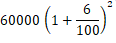== 60000(53/50)2
= 60000 × 53/50 × 53/50
= Rs. 67,416
After 1 year Vasudevan would get amount Rs. 67,416.

6. Arif took a loan of Rs.80,000 from a bank. If the rate of interest is 10% per annum, find the difference in amounts he would be paying after 1.1/2 years if the interest is:
(i) compounded annually.
(ii) compounded half yearly.

Solution

(i) Here, Principal (P) = Rs. 80,000
Time (n)= 1.1/2 years,
Rate of interest (R) = 10%
Amount for 1 year (A) ==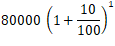== 80000 (11/10)1
= Rs. 88,000
Interest for 1/2 year = (88000×10×1)/(100×2) = Rs. 4,400
Total amount = Rs. 88,000 + Rs. 4,400 = Rs. 92,400

(ii) Here, Principal (P) = Rs.80,000,
Time (n) = 1.1/2 year = 3/2 years (compounded half yearly)
Rate of interest (R) = 10% = 5% (compounded half yearly)
Amount (A) ==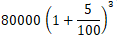== 80000(21/20)3
= 80000 × 21/20 × 21/20 × 21/20
= Rs. 92,610
Difference in amounts = Rs. 92,610 – Rs. 92,400 = Rs. 210

7. Maria invested Rs.8,000 in a business. She would be paid interest at 5% per annum compounded annually. Find:
(i) The amount credited against her name at the end of the second year.
(ii) The interest for the third year.

Solution

(i) Here, Principal (P) = Rs. 8000, Rate of Interest (R) = 5%, Time (n) = 2 years
Amount (A) ==== 8000(21/20)2
= 8000 × 21/20 × 21/20
= Rs. 8,820

(ii) Here, Principal (P) = Rs. 8000, Rate of Interest (R) = 5%, Time (n) = 3 years
Amount (A) ==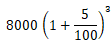== 8000(21/20)3
= 8000 × 21/20 × 21/20 × 21/20
= Rs. 9,261
Interest for 3rd year = A – P = Rs. 9,261 – Rs. 8,820 = Rs. 441

8. Find the amount and the compound interest on Rs.10,000 for 1.1/2 years at 10% per annum, compounded half yearly.
Would this interest be more than the interest he would get if it was compounded annually?

Solution

Here, Principal (P) = Rs. 10000,
Rate of Interest (R) = 10% = 5% (compounded half yearly)
Time (n) = 1.1/2 years = 3 years (compounded half yearly)
Amount (A) ===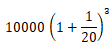= 10000 (21/20)3
= 10000 × 21/20 × 21/20 × 21/20
= Rs. 11,576.25
Compound Interest (C.I.) = A – P = Rs. 11,576.25 – Rs. 10,000 = Rs. 1,576.25
If it is compounded annually, then
Here, Principal (P) = Rs. 10000
Rate of Interest (R) = 10%,
Time (n) = 1.1/2years
Amount (A) for 1 year ==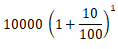=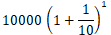= 10000(11/10)1
= 10000 × 11/10
= Rs. 11,000

Interest for 1/2 year = (11000 × 1 × 10)/(2×100) = Rs. 550
∴ Total amount = Rs. 11,000 + Rs. 550 = Rs. 11,550
Now, C.I. = A – P = Rs. 11,550 – Rs. 10,000 = Rs. 1,550
Yes, interest Rs. 1,576.25 is more than Rs. 1,550.

9. Find the amount which Ram will get on Rs.4,096, if he gave it for 18 months at 12.1/2% per annum, interest being compounded half yearly.

Solution

Here, Principal (P) = Rs. 4096,
Rate of Interest (R) = 12.1/2 = 25/2% = 25/4% (compounded half yearly)
Time (n)= 18 months = 1.1/2 years = 3 years (compounded half yearly)
Amount (A) ==== 4096(17/16)3
= 4096 × 17/16 × 17/16 × 17/16
= Rs. 4,913

10. The population of a place increased to 54,000 in 2003 at a rate of 5% per annum.
(i) Find the population in 2001.
(ii) What would be its population in 2005?

Solution

(i) Here, A2003 = Rs. 54,000,
R = 5%,
n = 2 years
Population would be less in 2001 than 2003 in two years.
Here population is increasing.
∴ A2003 =⇒ 54000 =⇒ 54000 =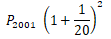⇒ 54000 = P2001(21/20)2
⇒ 54000 = P2001 ×21/20 × 21/20
⇒ P2001 = (54000 × 20 × 20)/(21 × 21) =48,979.5
⇒ P2001 = 48,980 (approx.)

(ii) According to question, population is increasing.
Therefore population in 2005,
A2015 == 54000 (1 + 5/100)2
= 54000(1+1/20)2
= 54000(21/20)2
= 54000 × 21/20 × 21/20
= 59,535
Hence population in 2005 would be 59,535.

11. In a laboratory, the count of bacteria in a certain experiment was increasing at the rate of 2.5% per hour. Find the bacteria at the end of 2 hours if the count was initially 5,06,000.

Solution

Here, Principal (P) = 5,06,000,
Rate of Interest (R) = 2.5%,
Time (n) = 2 hours
After 2 hours, number of bacteria,
Amount (A) == 506000(1+ 2.5/100)2
= 506000(1+25/1000)2
= 506000(1+1/40)2
= 506000(41/40)2
= 506000 × 41/40 × 41/40
= 5,31,616.25
Hence, number of bacteria after two hours are 531616 (approx.).

12. A scooter was bought at Rs.42,000. Its value depreciated at the rate of 8% per annum. Find its value after one year.

Solution

Here, Principal (P) = Rs. 42,000,
Rate of Interest (R) = 8%,
Time (n) = 1 years

Amount (A) = P ( 1 - R/100)n
= 42000(1 - 8/100)1
= 42000(1+ 2/25)1
= 42000 (27/25)1
= 42000 × 27/25
= Rs. 38,640
Hence, the value of scooter after one year is Rs. 38,640.

## NCERT Solutions for Class 8 Maths Chapter 8 Comparing Quantities

Chapter 8 NCERT Solutions can be used to solve difficult questions in a exercise. In this chapter, we are focusing on ratio, percentage and compound interest.

• When we compare two quantities of same kind by division, we have to form a ratio of the two quantities. Two equal ratios are said to form a proportion. In a proportion, product of extremes is equal to the product of the means.

• Another way of comparing quantities is to express them as percentages. Percentage means hundredth. It is the numerator of a fraction with denominator 100.

• The difference between the principal and the final amount is called Compound Interest.

There are total 3 exercises in the whole chapter which is going to help you in building problem solving skills and be aware of the concepts. Below you will get exerciseswise NCERT Solutions of Chapter 8 Comparing Quantities.

Studyrankers has prepared detailed and accurate NCERT Solutions of every questions through which a student can easily clear their doubts and get in depth insights. It will be very useful in preparation of the examinations and understanding the concepts properly.

### NCERT Solutions for Class 8 Maths Chapters:

 Chapter 1 Rational Numbers Chapter 2 Linear Equations in Variable Chapter 3 Understanding Quadrilaterals Chapter 4 Practical Geometry Chapter 5 Data Handling Chapter 6 Squares and Square Roots Chapter 7 Cubes and Cube Roots Chapter 9 Algebraic Expressions and Identities Chapter 10 Visualizing Solid Shapes Chapter 11 Mensuration Chapter 12 Exponents and Powers Chapter 13 Direct and Inverse Proportions Chapter 14 Factorization Chapter 15 Introduction to Graphs Chapter 16 Playing with Numbers

FAQ on Chapter 8 Comparing Quantities

#### What are the benefits of NCERT Solutions for Chapter 8 Comparing Quantities Class 8 NCERT Solutions?

Chapter 8 NCERT Solutions is best way through which one can revise all the topics and improve their learning behavior. It will be quite helpful in gaining good marks in the examinations.

#### A computer monitor is marked at ₹15600 and it is available for ₹12480. Find the discount given and discount per cent.

Marked price of the monitor = ₹15600
Sale price of the monitor = ₹12480
∴ Discount = [Marked price] – [Sale price]
= ₹15600 – ₹12480
= ₹15600 – ₹12480
∴ Discount per cent = ₹3120/₹15600 × 100%
= 20%.

#### In a survey of 50 students, 80% of students liked Science, and 20% of students liked Arts. How many numbers of students liked Science?

We know that 80% of students liked science in the survey of 50 students
∴ Number of students who liked Science = 80/100 × 50 = 40.

#### A table is marked at ₹940. If it is sold for ₹799, then find the discount per cent.

Marked Price = ₹ 940
Selling Price = ₹ 799
Discount = MP – SP = 940 – 799 = ₹141
∴ Discount percent = Discount/MP × 100
= 141/94 × 100 = 15%.